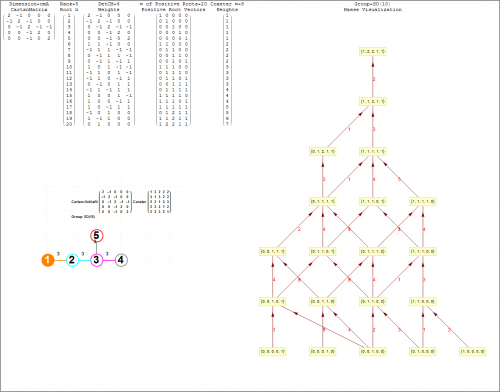# Visualizing E6 as a subgroup of E8 for a Leptoquark SUSY GUT?

MyToE was mentioned in a Luboš Motl blog post comment, so I thought I would throw out a few ideas and offer to help Luboš visualize the models/thinking around developing an E6 Leptoquark SUSY GUT.

Here are the E6 Dynkin related constructs, including the detail Hasse visualization.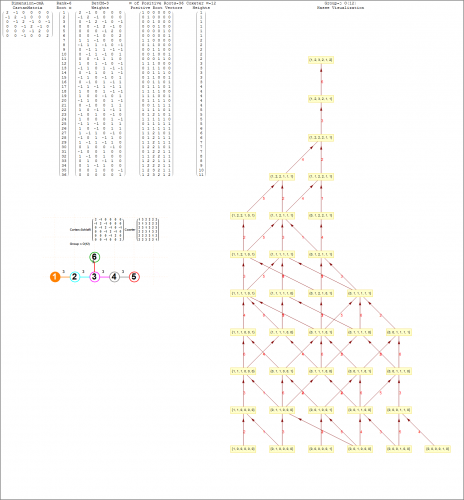For this post, I use Split Real Even (SRE) E8 vertices created by dot product of the 120 positive (and 120 negative roots) with the following Simple Roots Matrix (SRM), which generates the Cartan as well:This is a list of E6 vertices as a subset of SRE E8 by taking off the orthogonal right-side 2 dimensions (or Dynkin nodes in red) vertices with identical entries).This is an interesting E6 projection with basis vectors of:
X={2, -1, 1, 1/2, -1, -(1/2), 0, 0}
Y= {0, 0, Sqrt, Sqrt/2, Sqrt, Sqrt/2, 0, 0}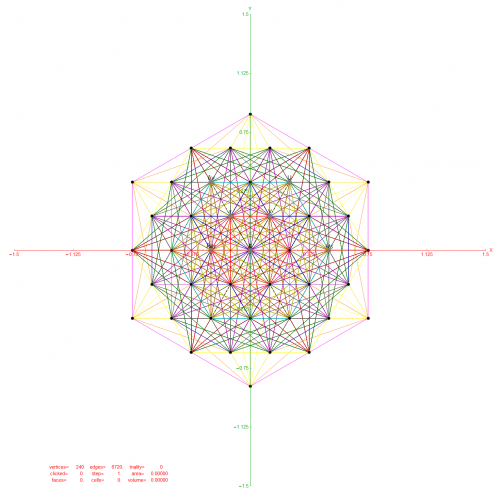Adding a third Z={0,0,Sqrt,Sqrt/2,Sqrt,Sqrt/2,0,0}, we get a 3D projection: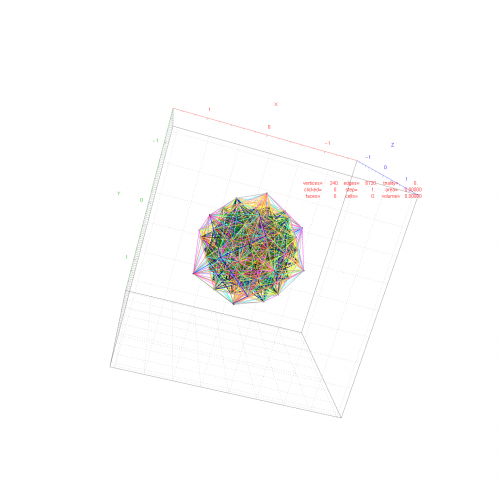Luboš> “the maximum subgroup we may embed to E6 is actually SO(10)×U(1).”

Since D5 (which is the same as E5 in terms of the Dynkin diagram topology) relates to SO(10), it is interesting to look at the complement of E6 vertices and the 40 D5 vertices contained in E6 (leaving 32):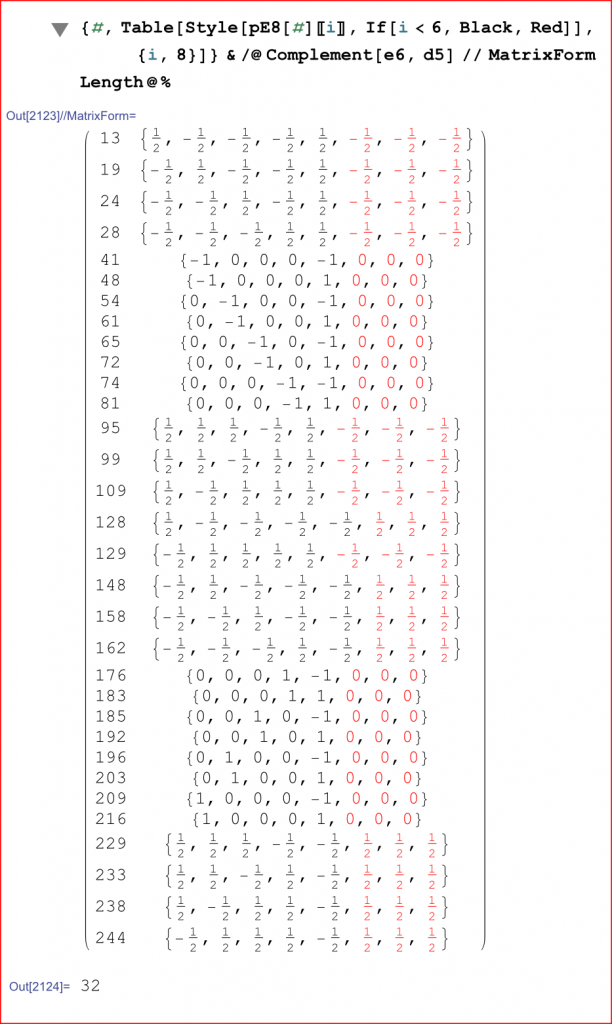Here is the D5 Dynkin and its Cartan algebra: